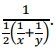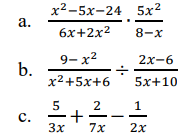# MA.912.AR.1.9Export Print
Apply previous understanding of rational number operations to add, subtract, multiply and divide rational algebraic expressions.

### Clarifications

Clarification 1: Instruction includes the connection to fractions and common denominators.
General Information
Subject Area: Mathematics (B.E.S.T.)
Strand: Algebraic Reasoning
Status: State Board Approved

## Benchmark Instructional Guide

### Terms from the K-12 Glossary

• Rational expression

### Vertical Alignment

Previous Benchmarks

Next Benchmarks

### Purpose and Instructional Strategies

In elementary and middle grades students perform operations with rational numbers, including fractions. In Math for College Algebra, they extend the work they did with operations with rational numbers to operations with rational expressions.
• Instruction includes the connection to fractions and common denominators. A rational expression, same as a fraction, can be written as a ratio. Instead of a ratio of two numbers, rational expressions are a ratio of two polynomial expressions.
• The properties of fractions can be applied to rational expressions.
• Simplifying rational expressions
• Factor the numerator and denominator and extract common factors.
• Multiply rational expressions
• It may be helpful to first, factor the numerator and the denominator then, multiply the numerators and multiply the denominators.
• Divide rational expressions
• Students can multiply the first expression by the reciprocal of the second expression and follow the multiplication steps.
• Adding and subtracting rational expressions
• Students can find a common denominator and then add or subtract the numerators.

### Common Misconceptions or Errors

• When simplifying rational expressions students cancel out terms instead of factors. Remind students to always factor before simplifying.
• When simplifying out the entire numerator students forget to write 1 as the numerator.
• When multiplying rational expressions students cross multiply instead of multiplying across.
• When adding and subtracting rational expressions students tend to forget to find a common denominator.
• When adding and subtracting rational expressions students mistakenly follow the steps of multiplication (i.e., add across the denominators and/or add across the numerators).

• The U.S. Department of Energy keeps track of fuel efficiency for all vehicles sold in the United States. Each car has two fuel economy numbers, one measuring efficient for city driving and one for highway driving. For example, a 2012 Volkswagen Jetta gets 29.0 miles per gallon (mpg) in the city and 39.0 mpg on the highway.
• Many banks have “green car loans” where the interest rate is lowered for loans on cars with high combined fuel economy. This number is not the average of the city and highway economy values. Rather, the combined fuel economy (as defined by the federal Corporate Average Fuel Economy standard) for $x$ mpg in the city and $y$ mpg on the highway, is 1 computed as• Part A. What is the combined fuel economy for the 2012 Volkswagen Jetta? Give your answer to three significant digits.
• Part B. For most conventional cars, the highway fuel economy is 10 mpg higher than the city fuel economy. If we set the city fuel economy to be $x$ mpg for such a car, what is the combined fuel economy in terms of $x$? Write your answer as a single rational function $\frac{\text{a(x)}}{\text{b(x)}}$.
• Part C. Rewrite your answer from Part B in the form of $q$($x$) + $\frac{\text{r(x)}}{\text{b(x)}}$ where and $q$($x$), $r$($x$) and $b$($x$) are polynomials and the degree of $r$($x$) is less than the degree of $b$($x$).
• Part D. Use your answer in Part C to conclude that if the city fuel economy, $x$, is large, then the combined fuel economy is approximately $x$ + 5.

• Tamisha walked 4 miles to the store to buy a new bike at an average speed of $x$ miles per hours. She returned home riding her bike and her average speed was 2 miles faster than walking.
• Part A. Write an expression for the time Tamisha takes to get to the store.
• Part B. Write an expression for the time Tamisha takes to get home from the store.
• Part C. Write an expression for the total time she spent on both trips.
• Part D. If Tamisha spent 3 hours on both trips, what is Tamisha’s average walking speed.

### Instructional Items

Instructional Item 1 (MTR.3.1
• Perform the operation(s) on the rational expression. Write your answer as single rational expression.*The strategies, tasks and items included in the B1G-M are examples and should not be considered comprehensive.

## Related Courses

This benchmark is part of these courses.
1200330: Algebra 2 (Specifically in versions: 2014 - 2015, 2015 - 2022, 2022 and beyond (current))
1200340: Algebra 2 Honors (Specifically in versions: 2014 - 2015, 2015 - 2022, 2022 and beyond (current))
1200400: Foundational Skills in Mathematics 9-12 (Specifically in versions: 2014 - 2015, 2015 - 2022, 2022 and beyond (current))
7912095: Access Algebra 2 (Specifically in versions: 2016 - 2018, 2018 - 2019, 2019 - 2022, 2022 and beyond (current))
1200710: Mathematics for College Algebra (Specifically in versions: 2022 and beyond (current))

## Related Access Points

Alternate version of this benchmark for students with significant cognitive disabilities.
MA.912.AR.1.AP.9: Apply previous understanding of rational number operations with common denominators to add and subtract rational expressions.

## Related Resources

Vetted resources educators can use to teach the concepts and skills in this benchmark.

## Lesson Plan

A Rational Representation:

Students will tackle a real-world situation regarding starting a business that requires a rational equation to evaluate the plan. Students will determine a method and set of steps for solving rational equations and then revisit the original scenario and solve using the new method they have synthesized. Students will also explore, through collaborative learning structures, the concept of extraneous solutions.

Type: Lesson Plan

## Student Resources

Vetted resources students can use to learn the concepts and skills in this benchmark.

## Parent Resources

Vetted resources caregivers can use to help students learn the concepts and skills in this benchmark.